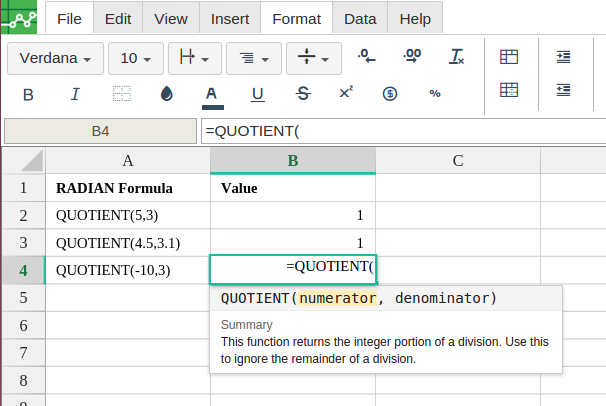# QUOTIENT

## Definition

Returns one number divided by another, without the remainder.

#### Sample Usage

`QUOTIENT(4,2)`

`QUOTIENT(A2,B2)`

#### Syntax

`QUOTIENT(dividend, divisor)`

• `dividend` - The number to be divided.

• `divisor` - The number to divide by (cannot equal `0`).

`SUM`: Returns the sum of a series of numbers and/or cells.

`PRODUCT`: Returns the result of multiplying a series of numbers together.

`MULTIPLY`: Returns the product of two numbers. Equivalent to the `*` operator.

`MINUS`: Returns the difference of two numbers. Equivalent to the `-` operator.

`DIVIDE`: Returns one number divided by another. Equivalent to the `/` operator.

`ADD`: Returns the sum of two numbers. Equivalent to the `+` operator.

####Error.

User does not have sufficient privileges to access this Content